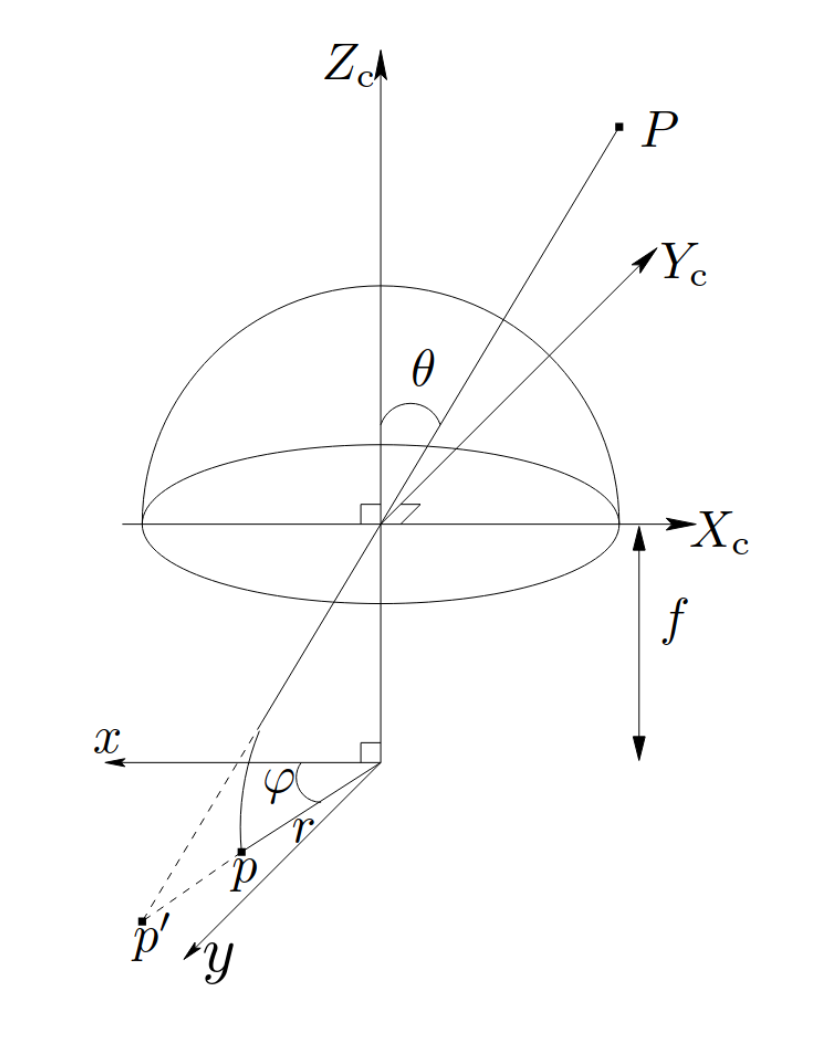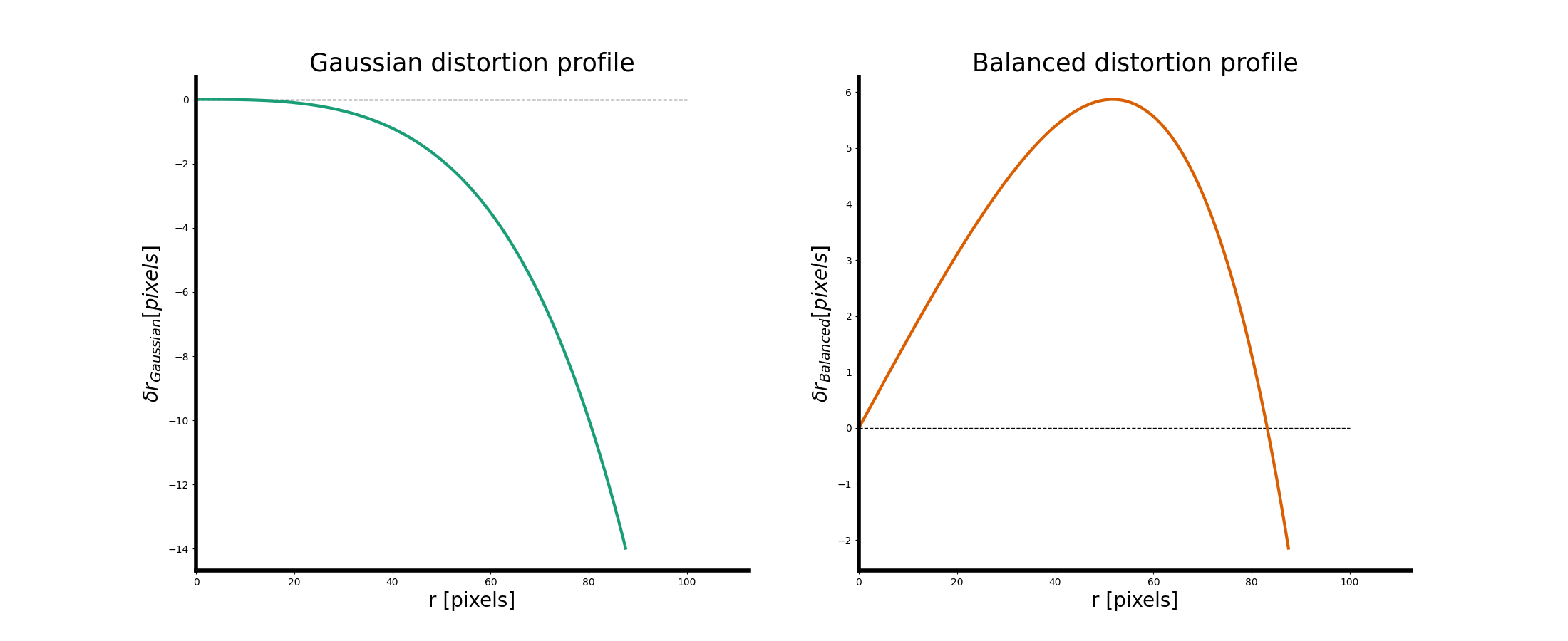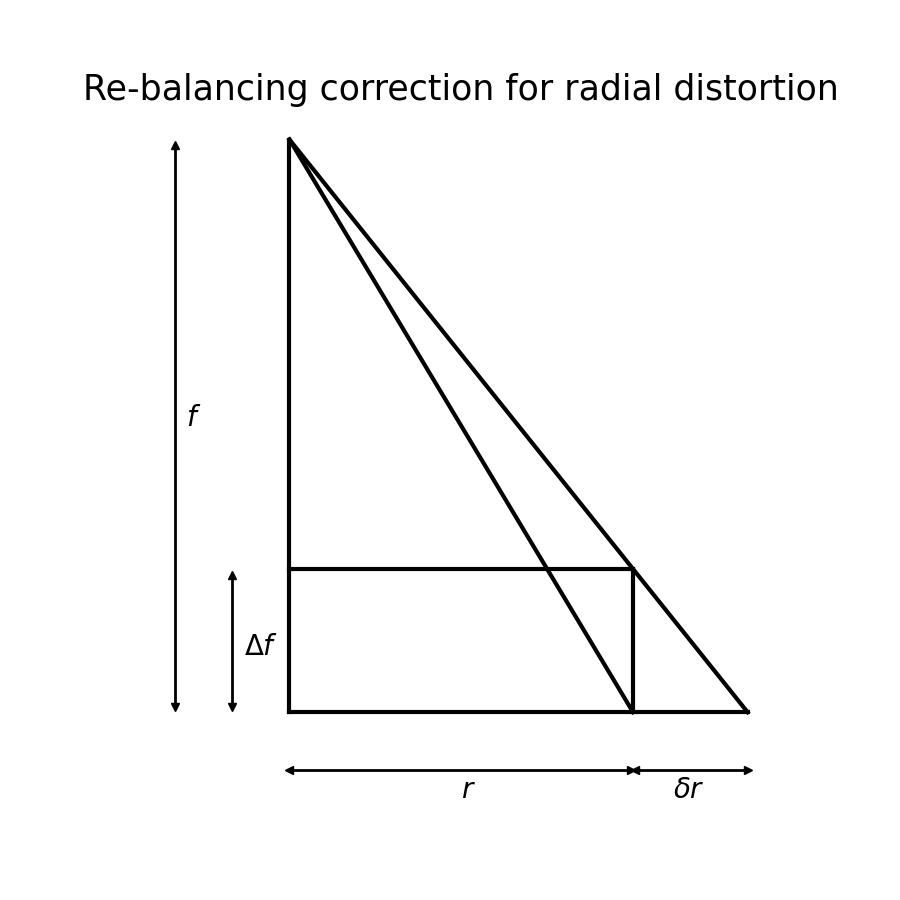< Back to Blog

# Camera Modeling: Exploring Distortion and Distortion Models, Part II

###### August 9, 2021
Tutorial

In Part I, we described in detail the variety of lens distortion types that impact vision system performance and calibration. But what do you do when you encounter lens distortion? You correct it with a distortion model. Despite the proliferation and prevalence of cameras and vision-enabled devices over the past century or so, there have been two primary distortion models that have gained widespread adoption to provide correction. We'll go over these, and dive into the math and approach to ground you in these techniques.

## Common Distortion Models

While there are more models than what is described here, the industry has largely standardized on the following two distortion models.

Brown-Conrady distortion is probably what most think of as the "standard" radial and tangential distortion model. This model finds its roots in two | documents, authored by Brown and Conrady. The documents are quite old and date up to a century ago, but still form the foundation of many of the ideas around characterizing and modeling distortion today!

This model characterizes radial distortion as a series of higher order polynomials:

$$r = \sqrt{x^2 + y^2}$$

$$\delta r= k_1 r^3 + k_2 r^5 + k_3 r^7 + ... + k_n r^{n+2}$$

In practice, only the $$k_1$$ through $$k_3$$ terms are typically used. For cameras with relatively simple lens assemblies (e.g. only contain one or two lenses in front of the CMOS/CCD sensor), it is often sufficient to just use the $$k_1$$ and $$k_2$$ terms.

To relate this back to our image coordinate system (i.e. $$x$$ and $$y$$), we usually need to do some basic trigonometry:

$$\delta x_r = \sin(\psi) \delta r = \frac{x}{r} (k_1r^3 + k_2r^5 + k_3r^7)$$

$$\delta y_r = \cos(\psi) \delta r = \frac{y}{r} (k_1r^3 + k_2r^5 + k_3r^7)$$

💡 The original documents from Brown and Conrady did not express $$\delta r$$ in just these terms, and in fact the original documents state everything in terms of "de-centering" distortion broken into radial and tangential components. Symmetric radial distortion as expressed above is a mathematical simplification of the overall power-series describing the radial effects of a lens. The formula we use above is what we call the "Gaussian profile" of Seidel distortion.

Wikipedia has a good summary of the history here, but the actual formalization is beyond the scope of what we want to cover here.

Tangential distortion, as characterized by the Brown-Conrady model, is often simplified into the following $$x$$ and $$y$$ components. We present these here first as they are probably what most are familiar with:

$$\delta x_t = p_1(r^2 + 2x^2) + 2p_2xy$$

$$\delta y_t = p_2(r^2 + 2y^2) + 2p_1xy$$

This actually derives from an even-power series much like the radial distortion is an odd-power series. The full formulation is a solution to the following:

$$\delta t = P(r) cos(\psi - \psi_0)$$

Where $$P(r)$$ is our de-centering distortion profile function, $$\psi$$ is the polar angle of the image plane coordinate, and $$\psi_0$$ is the angle to the axis of maximum tangential distortion (i.e. zero radial distortion). Expanding this into the general parameter set we use today is quite involved (read the original Brown paper!), however this will typically take the form:

$$\delta x_t = [p_1(r^2 + 2x^2) + 2p_2xy](1 + p_3r^2 + p_4r^4 + p_5r^6 + ...)$$

$$\delta y_t = [p_2(r^2 + 2y^2) + 2p_1xy](1 + p_3r^2 + p_4r^4 + p_5r^6 + ...)$$

Because tangential distortion is usually small, we tend to approximate it using only the first two terms. It is rare for de-centering to be so extreme that our tangential distortion requires higher order terms because that would mean that our lens is greatly de-centered relative to our image plane. In most cases, one might ask if their lens should simply be re-attached in a more appropriate manner.

## Kannala-Brandt

Almost a century later (2006, from the original Conrady paper in 1919), Juho Kannala and Sami Brandt published their own paper on lens distortions. The main contribution of this paper adapts lens distortion modeling to be optimized for wide-angle, ultra wide-angle, and fish-eye lenses. Brown & Conrady's modeling was largely founded on the physics of Seidel aberrations, which were first formulated around 1867 for standard lens physics of the time, which did not include ultra wide and fish-eye lenses.

The primary difference that most folks will notice using this model lies in symmetric radial distortion. Rather than characterizing radial distortion in terms of how far a point is from the image centre (the radius), Kannala-Brandt characterizes distortion as a function of the incidence angle of the light passing through the lens. This is done because the distortion function is smoother when parameterized with respect to this angle ($$\theta$$), which makes it easier to model as a power-series:

$$\theta = \arctan(\frac{r}{f})$$

$$\delta r = k_1\theta + k_2\theta^3 + k_3\theta^5 + k_4\theta^7 + ... + k_n\theta^{n+1}$$

Above, we've shown the formula for $$\theta$$ when using perspective projection, but the main advantage of the Kannala-Brandt model is that it can support different kinds of projection by swapping our formula for $$\theta$$, which is what makes the distortion function smoother for wide-angle lenses. See the following figure, shared here from the original paper, for a better geometric description of $$\theta$$:Projection of quantities used in Kannala-Brandt distortion. Shared from the original paper, "A Generic Camera Model and Calibration Method for Conventional, Wide-angle, and Fish-eye Lenses," by J. Kannala and S. Brandt

Kannala-Brandt also aims to characterize other radial (such as asymmetric) and tangential distortions. This is done with the following additional parameter sets:

$$\delta r_{other} = (l_1\theta + l_2\theta^3 + l_3\theta^5) (i_1 \cos(\psi) + i_2 \sin(\psi) + i_3 \cos(2\psi) + i_4 \sin(2\psi) + ...)$$

$$\delta t = (m_1\theta + m_2 \theta^3 + m_3 \theta^5)(j_1\cos(\psi) + j_2\sin(\psi) + j_3 \cos(2\psi) + j_4 \sin(2\psi) + ...)$$

Overall, this results in a 23 parameter model! This is admittedly overkill, and the original paper claims as much. These models, unlike the symmetric radial distortion, are an empirical model derived by fitting an N-term Fourier series to the data being calibrated. This is one way of characterizing it, but over-parameterizing our final model can lead to poor repeatability of our final estimated parameters. In practice, most systems will characterize Kannala-Brandt distortions purely in terms of the symmetric radial distortion, as that distortion is significantly larger in magnitude and will be the leading kind of distortion in wider-angle lenses.

Overall, Kannala-Brandt has been chosen in many applications for how well it performs on wide-angle lens types. It is one of the first distortion models to successfully displace the Brown-Conrady model after decades.

## Gaussian vs. Balanced Profiles

An astute reader of this article might be thinking right now: "Hey, <library-that-I-use> doesn't use these exact models! That math seems off!" You would mostly be correct there: this math is a bit different from how many popular libraries will model distortion (e.g. OpenCV). Using Brown-Conrady as an example, you might see symmetric radial distortion formulated as so:

$$\delta r = r + k_1 r^3 + k_2 r^5 + k_3 r^7$$

$$\theta = \arctan(r)$$

$$\delta r = \theta + k_1\theta^3 + k_2\theta^5 + k_3\theta^7 + k_4\theta^9$$

This probably seems quite confusing, because all these formulae are a bit different from the papers presented in this article. The main difference here is that the distortions have been re-characterized using what is referred to in photogrammetry as the Balanced Distortion Profile. Up until now, we have been presenting distortions using what is referred to in photogrammetry as the Gaussian Distortion Profile.Example comparing two equivalent distortion models. The left describes the Gaussian distortion profile, whereas the right hand side describes a Balanced distortion profile. The distortions are effectively the same, but the model is transformed in the balanced profile scenario. Notice the two different scales in the left and right figures.

As can be seen in the figure above, the profile and maximum magnitude of distortion are fundamentally different in the above cases. More specifically, the balanced profile is one way to limit the maximum distortion of the final distortion profile, while still correcting for the same effects. So how does one go from one representation to the other? For Brown-Conrady, this is done by scaling the distortion profile by a linear correction:

$$\delta r_{Balanced} = k_0' r + k_1' r^3 + k_2 'r^5 + k_3'r^7$$

$$\delta r_{Balanced} = \frac{\Delta f}{f} r + \left(1 + \frac{\Delta f}{f} \right)(k_1r^3 + k_2r^5 + k_3r^7)$$

Note that this linear relationship is derived by similar triangles for Brown-Conrady distortions by the relationships shown in the following figure:A set of similar triangles representing the linear correction done to re-balance the Gaussian distortion profile. Re-balancing is used to set the distortion to zero at some radius, or to reconfigure the distortion profile to characterize itself for a virtual focal length.

Given that, why does this discrepancy exist? There are a few reasons this could be used. Historically, the balanced profile was used because distortion was measured manually through the use of mechanical stereoplotters. These stereoplotters had a maximum range in which they could move mechanically, so limiting the maximum value of distortion made practical sense.

But what does that mean for today? Nowadays with the abundance of computing resources it is atypical to use mechanical stereoplotters in favour of making digital measurements on digital imagery. There doesn't seem to be a paper trail for why this decision was made, so it could just be a historical artefact. However, doing the re-balancing has some advantages:

1. By parameterizing the model with $$\frac{\Delta f}{f} = 1$$, as OpenCV does, it makes the math and partial derivatives for self-calibration somewhat easier to implement. This is especially true when using the Kannala-Brandt model, since doing this removes the focal length term from $$\theta = \arctan(r),$$ which means that there is one less partial derivative to compute.
2. If you keep your entire self-calibration pipeline in units of "pixels" as opposed to metric units like millimetres or micrometres, then the Gaussian profile will produce values for $$k_1, k_2, k_3$$ that are relatively small. On systems that do not have full IEEE-754 floats (specifically, systems that use 16-bit floats or do not support 64-bit floats at all), this could lead to a loss in precision. Some CPU architectures today do lack full IEEE-754 support (armel, I'm looking at you), so the re-balancing could have been a consideration for retaining machine precision without adding any specialized code. There is no difference in the final geometric precision or accuracy of the self-calibration process as a result of the re-balancing, as it is just a different model.
3. All of the above math is derived from basic lens geometry, and presents a problem if one has to choose a focal length $$f$$ between $$f_x$$ and $$f_y$$! So if you're already using $$f_x$$ and $$f_y$$, removing all focal length terms from e.g. Kannala-Brandt distortions may appear to save you from having to make poor approximations of the quantities involved.

Despite these reasons, there is also one very important disadvantage. Notably: the balanced profile with Brown-Conrady distortions introduces a factor of $$( 1 + \frac{\Delta f}{f})$$ into the determination of $$k_1$$ through $$k_3$$. This may not seem like much, but it means that we are introducing a correlation in the determination of these parameters with our focal length. If one chooses a model where $$f_x$$ and $$f_y$$ are used, then the choice in parameterization will make the entire calibration unstable, as small errors in the determination of any of these parameters will bleed into every other parameter. This is one kind of projective compensation, and is another reason for why our last article on the subject suggested not to use this parameterization.

It might also seem that with the Kannala-Brandt distortion model, we simplify the math by cancelling out $$f$$ from our determination of $$\theta$$. This is true, and it will make the math easier, and remove a focal length term from our determination of the Kannala-Brandt parameters. However, if one chooses a different distortion projection, e.g. orthographic for a fish-eye lens:

$$\theta_{Gaussian} = \arcsin \left(\frac{r}{f} \right)$$

$$\theta_{Balanced} = \arcsin(r)$$

Then one will quickly notice that the limit of $$\theta_{Balanced}$$  is not well defined as $$r \rightarrow \infty$$, and gives us a value of $$(-i)\infty$$. As we've tried to separate our focal length from our parameters, we've ended up wading into the territory of complex numbers! Since our max radius and focal length are often proportional, $$\theta_{Gaussian}$$ does not suffer from the same breakdown in values at the extremes.

💡 It may seem like Kannala-Brandt is a poor choice of model as a result of this. For lenses with a standard field-of-view, the Brown-Conrady model with the Gaussian profile does a better job of determining the distortion without introducing data correlations between the focal length and distortion parameters.

However, the Brown-Conrady model does not accommodate distortions from wide-field-of-view lenses very well, such as when using e.g. fisheye lenses. This is because they were formulated on the basis of Seidel distortions, which do not operate the same way as the field-of-view increases past 90°.

The Kannala-Brandt model, while introducing some correlation between our determination of $$f$$ and our distortion coefficients $$k_1$$ through $$k_4$$, does a better job of mapping distortion with stereographic, orthographic, equidistance, and equisolid projections. As with anything in engineering there are trade-offs, and despite the extra correlations, the Kannala-Brandt model will still often provide better geometric precision of the determined parameters compared to the Brown-Conrady model in many of these scenarios.

As can be seen, chasing simplicity in the mathematical representations is one way in which our choice of model can result in unstable calibrations, or nonsensical math. Given that we want to provide the most stable and precise calibrations possible, we lean towards favouring the Gaussian profile models where possible. It does mean some extra work to make sure the math is correct, but also means that by getting this right once, we can provide the most stable and precise calibrations ever after.

## Wrapping Up

We've explored camera distortions, some common models for camera distortions, and explored the ways in which these models have evolved and been implemented over the past century. The physics of optics has a rich history, and there's a lot of complexity to consider when picking a model.

Modeling distortion is one important component of the calibration process. By picking a good model, we can reduce projective compensations between our focal length $$f$$ and our distortion parameters, which leads to numerical stability in our calibration parameters.

Fitting in with our previous camera model, we can formulate this in terms of the collinearity relationship (assuming Brown-Conrady distortions):

$$\begin{bmatrix} x_i \\ y_i \end{bmatrix} = \begin{bmatrix} f \cdot X_t / Z_t \\ f \cdot Y_t / Z_t \end{bmatrix} + \begin{bmatrix} a_1 x_i \\ 0 \end{bmatrix} + \begin{bmatrix} x_i (k_1 r^2 + k_2r^4+k_3r^6) \\ y_i(k_1r^2 + k_2r^4 + k_3r^6) \end{bmatrix} + \\\ \begin{bmatrix} p_1(r^2 + 2x_i^2) + 2 p_2 x_i y_i \\ p_2(r^2 + 2y_i^2) + 2 p_1 x_i y_i \end{bmatrix}$$

or, with Kannala Brandt distortions:

$$\begin{bmatrix} x_i \\ y_i \end{bmatrix} = \begin{bmatrix} f \cdot X_t / Z_t \\ f \cdot Y_t / Z_t \end{bmatrix} + \begin{bmatrix} a_1 x_i \\ 0 \end{bmatrix} + \begin{bmatrix} \frac{x_i}{r} (k_1 \theta + k_2\theta^3+k_3\theta^5 + k_4\theta^7) \\ \frac{y_i}{r} (k_1 \theta + k_2\theta^3+k_3\theta^5 + k_4\theta^7) \end{bmatrix}$$

Did we get too far into the weeds with this? Never fear, Tangram Vision has you covered. If you're worried about the best model for your camera and would rather do anything else, check out the Tangram Vision SDK! We're building perception tools today that lift the burden off your shoulders.

Update: Part III of this series makes the distinction between forward and inverse models, clarifies terms, and explains how we apply distortion models in-house. Read on at https://www.tangramvision.com/blog/camera-modeling-exploring-distortion-and-distortion-models-part-iii

## Accelerating Perception

The Tangram Vision Platform lets perception teams develop and deploy faster.